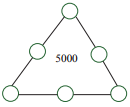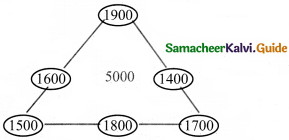Students can download 4th Maths Term 1 Chapter 2 Numbers Ex 2.6 Questions and Answers, Notes, Samacheer Kalvi 4th Maths Guide Pdf helps you to revise the complete Tamilnadu State Board New Syllabus, helps students complete homework assignments and to score high marks in board exams.

## Tamilnadu Samacheer Kalvi 4th Maths Solutions Term 1 Chapter 2 Numbers Ex 2.6

Question 1.
Fill in the boxes.
(i) 4634 + ______ = 4634
4634 + 0 = 4634

(ii) 2134 + 1 = ______
2134 + 1 = 2135(iii) 5439 + 0 = ______
5439 + 0 = 5439

(iv) 1435 + 1923 + = 1923 + ______
1435 + 1923 + = 1923 + 1435

(v) 3457 + _______ = 3458
3457 + 1 = 3458

Question 2.
Question 1.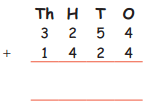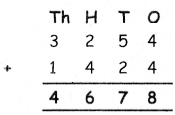Question 2.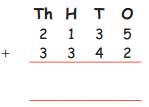Question 3.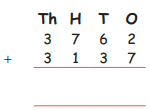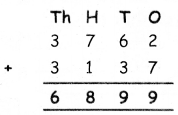Question 4.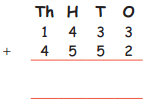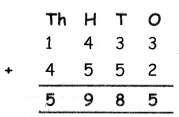Question 3.
Add: 2713 + 104 + 1172 + 6010
2713 + 104 + 1172 + 6010 = 9999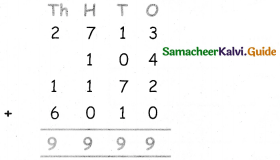Question 4.
A man visited a furniture shop. He bought a bed for ₹ 2100, a dining table for ₹ 3500, and six chairs for ₹ 4200. How much money did he pay to the shop keeper?
Cost of bed = ₹ 2100
Cost of dining table = ₹ 3500
Cost of six chairs = ₹ 4200
Total = ₹ 2100 + ₹ 3500 + ₹ 4200 = ₹ 9800Question 5.
Create word problem for the addition facts given below.
(a) 3094 + 7923 = 11,017
(b) 8309 = 2309 + _____
(a) 3094 + 7923 = 11,017
Geetha has 3094 Tomatos and Sandy has 7923 Tomatos. Then what is the total number of Tornatos.

(b) 8309 = 2309 + _____
If Vipin adds 6000 with 2309 then what will be the sum?
2309 + 6000 = 8309.Question 6.
Create addition stories using the pictures and numbers given below.
(a)What is sum of the price two bags?

(b)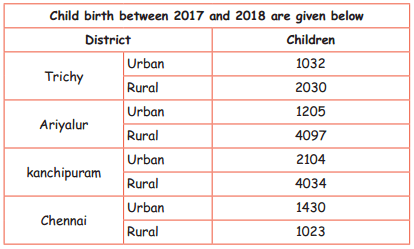How many childerns are there in the Urban?
How many childerns are there in Rural?Question 7.
Fill in the circles using 1400, 1500, 1600, 1700, 1800 and 1900 so that the three numbers along each line add up to 5000.